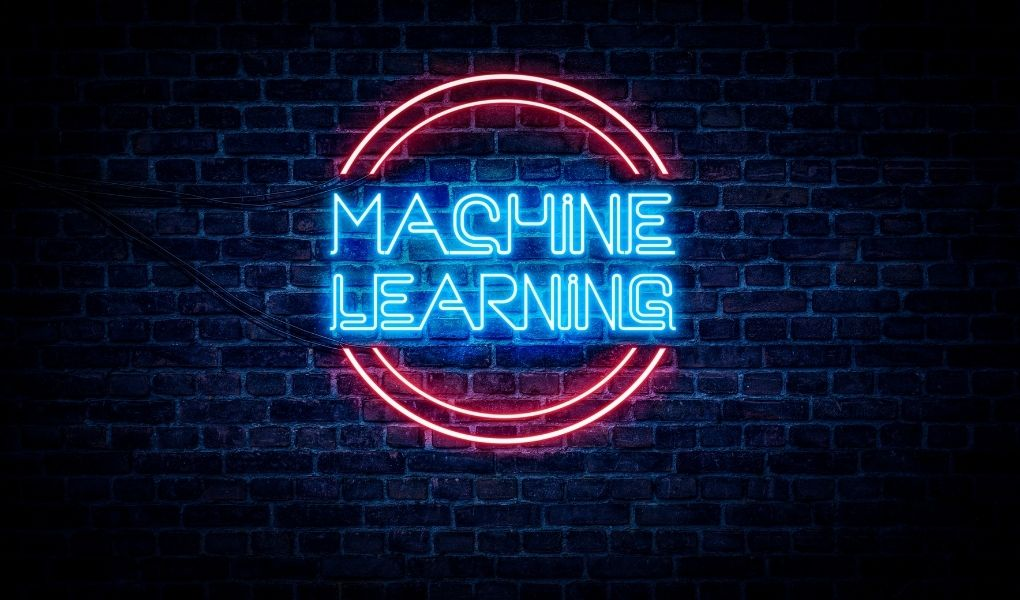Latest:
TECHNOLOGY

# Top 5 Most Popular Machine Learning AlgorithmsMachine learning is a whole universe, and each algorithm in this area is a galaxy with its characteristics, applications, and optimization strategies. Today we will briefly analyze the most popular Machine Learning algorithms and see where and when they should be applied.

## 1. Linear regression

What is it?: If you have a sequence of numbers [100, 200, 300, 400, 500, x], linear regression will easily predict that x should be 600. This algorithm predicts simple linear data relationships and values ​​within trends.

Where to apply: Simple analysis and prediction of linear data and trends, Working with variables that linearly depend on one, two, or three other variables.

Pros: A very simple and intuitive machine learning model. Easy to implement, fast to work.

Minuses: It only works well on very simple dependencies. Poorly predicts complex dependencies.

## 2 .Logistic regression

What is it?:The simplest classification algorithm came to us from the world of statistics. Allows dividing into two classes simple objects, whose state is controlled and described by a few parameters.

Logistic regression answers a number in the range from 0 to 1. If the number is below a certain threshold, then the object belongs to the first class of objects, and if it is higher, then to the second. The calibration of the threshold value for dividing objects into classes is selected during the calibration of the algorithm.

Where to apply:Where a simple classification of a small number of objects into a small number of classes is required

Pros: Speed ​​and clarity.

Minuses: It can only classify relatively simple objects. Not suitable for dividing objects into several classes, the answer of this algorithm is, in fact, a binary signal of the “yes-no” type.

## 3. K-means

What is it? : This machine-learning algorithm can group objects based on similarity. It scatters many objects into several classes with approximately similar properties.

Where to apply : Search for patterns, classification of objects according to several parameters. Working with objects that a set of variables can describe.

Pros: Consistency, simplicity, reliability, high speed of work.

Minuses: Poorly distinguishes objects that are too similar. Sometimes it can incorrectly determine the class of objects even after carefully calibrating the model.

## 4. Support Vector Machine

What is it?: Another classifier, radically different in its structure from the previous one, tries to build such a line to most accurately distinguish between different types of objects.

Where to apply?: Classification of objects.

Pros: Ease of implementation, work with multidimensional data.

Minuses: Like the K-Means algorithm, it can easily confuse objects that are similar to objects of another class.

## 5. Bayesian Classifier

What is it?: As the name suggests, this algorithm determines the class to which an object belongs. The classification mechanisms are based on calculating the probability of an object to a particular type of data. This method came to us from the world of statistics.

Where to apply?: In classification problems, of course. For example, the classic task is to tell if an email is spam or not.

Pros: Ease of implementation.

Minuses: The probabilistic approach has difficulty in classifying complex objects.

Also Read: Java & Node.js Frameworks For Microservices: Which Are Suitable